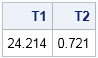I love working with SAS Technical Support because I get to see real problems that SAS customers face as they use SAS/IML software. The other day I advised a customer how to improve the efficiency of a computation that involved multiplying large matrices. In this article I describe an important efficiency tip: Never multiply with a diagonal matrix.

Here is the scenario. The customer needed to compute the matrix Z, which is the symmetric matrix product
Z = W1/2 B R B′ W1/2
where

• W = diag(d) is an N x N diagonal matrix
• B is an N x p matrix
• B′ is the transpose of B
• R is a p x N symmetric matrix. (The symmetry of R isn't exploited in this article.)
In the customer's scenario, N and p were large. Roughly, N = 10,000 and p = 600. The matrix computation was taking a long time, and because the computation was inside a simulation loop, the entire program required many hours to run.

### The brute force approach

The customer implemented the formula in the natural way. Let's time how long the straightforward computation takes. (I am using SAS 9.4, which uses multithreaded matrix multiplication. If you are using SAS 9.3, you might want to use N = 5000.) Since the contents of the matrices don't matter, I'll create random elements. As I've shown in a previous blog post, you can use the SQRVECH function to create the symmetric matrix R:

```proc iml; N = 10000; p = 600; /* define matrices */ d = j(N,1,1); /* d is N x 1 */ B = j(N,p,1); /* B is N x p */ v = j(p*(p+1)/2, 1, 1); /* allocate vector */ /* fill with random uniform numbers in (0,1) */ call randgen(d,"Uniform"); call randgen(B,"Uniform"); call randgen(v,"Uniform"); R = sqrvech(v); /* create symmetric p x p matrix */   /* straightforward (but slow) computation */ t0 = time(); W = diag(d); /* N x N diagonal matrix */ Z1 = sqrt(W) * B * R * B` * sqrt(W); T1 = time() - t0;```

On my computer, the naive computation with N = 10000 takes about 24 seconds. I think we can do better with a few small modifications.

### Never multiply with a diagonal matrix

The time required to compute this matrix expression can be dramatically shortened by implementing the following improvements:

• W is a diagonal matrix. Therefore computation sqrt(W) * B multiplies the ith row of B by the ith element of the diagonal of W1/2. You can compute this expression more efficiently by using elementwise multiplication (#) operator, as I showed in an article about converting a correlation matrix into a covariance matrix. The simpler expression is sqrt(d) # B, which also avoids forming the huge N x N diagonal matrix, W, and avoids taking the square-root of N2 elements, most of which are 0.
• The expression sqrt(W) * B appears twice. The expression appears at the beginning of the formula, and the transpose of the expression appears at the end of the formula. Whenever you see a computation repeated twice, you should consider creating a matrix to hold the intermediate result, such as C = sqrt(d) # B.

If you implement these two improvements, the computation executes much quicker. On my computer it now takes less than a second:

```free W; /* release the W memory */ /* avoid forming diag(d) and store temporary result */ t0 = time(); C = sqrt(d) # B; Z2 = C * R * C`; T2 = time() - t0; print T1 T2;```When you use a huge N x N diagonal matrix to multiply B, most of the time is spent multiplying the off-diagonal elements, which are zero. The naive approach multiplies (and adds) about 100 million zeros! The elementwise multiplication does not multiply any zeros. Getting rid of the diagonal matrix makes a major difference in the speed of the computation, and leads to the following efficiency tip:
Tip: Never, ever, multiply with a large diagonal matrix! Instead, use elementwise multiplication of rows and columns.

Specifically, if d is a column vector:

• Instead of diag(d) * A, use d # A to multiply the ith row of A by the ith element of d.
• Instead of A * diag(d), use A # d` to multiply the jth column of A by the jth element of d.